Custom SearchADDITION It has been shown that in adding like fractions we add the numerators. In adding unlike fractions,  the fractions must first be changed so that they have common denominators. We apply these same rules in adding mixed numbers. It will be remembered that a mixed number is an indicated sum. Thus,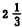is really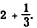. Adding can be done in any order. The following examples will show the application of these rules : EXAMPLE: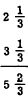This could have been written as follows: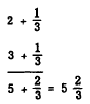EXAMPLE: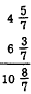Here we change 8/7 to the mixed number 1 1/7. ThenEXAMPLE: Add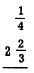We first change the fractions so that they are like and have the least common denominator and then proceed as before,EXAMPLE: Add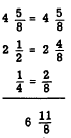Since 11/8 equals 1 3/8, the final answer is found as follows:Practice problems. Add, and reduce the sums to simplest terms: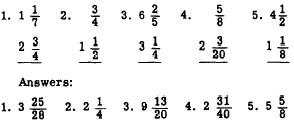The following example demonstrates a practical application of addition of fractions: EXAMPLE: Find the total length of the piece of metal shown in figure 4-5 (A). SOLUTION: First indicate the sum as follows:Changing to like fractions and adding numerators,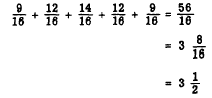Total length is 3 1/2 inches Practice problem. Find the distance from the center of the first hole to the center of the last hole in the metal plate shown in figure 4-5 (B). Answer 2 7/16 inches